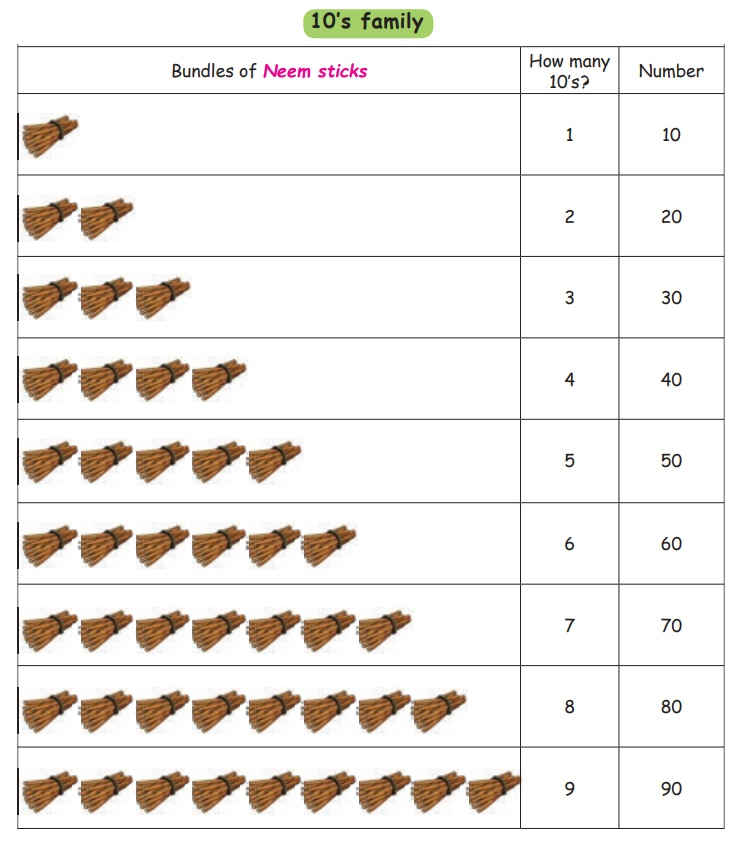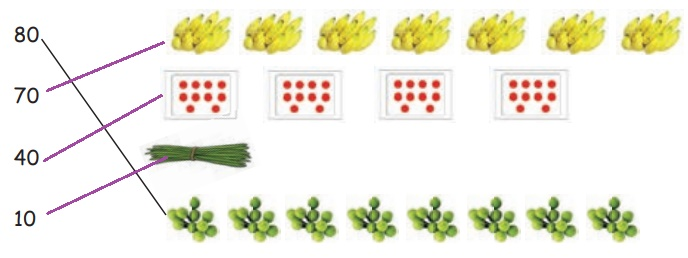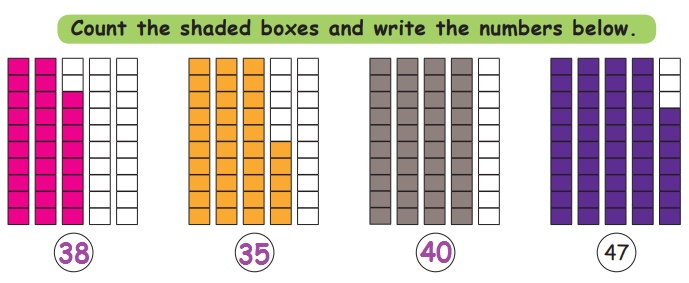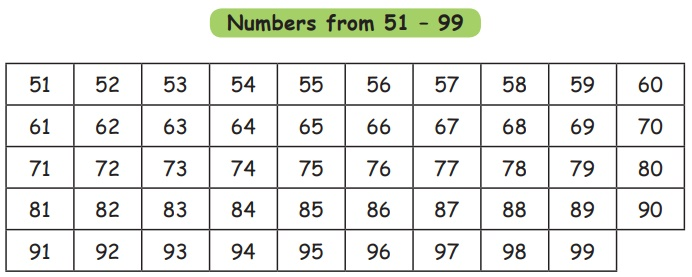Home | | Maths 1st Std | Numbers from 21 to 99

# Numbers from 21 to 99

Keywords: Numbers, Forward, Backward, Skip count

Numbers from 21 to 99

Keywords: Numbers, Forward, Backward, Skip count

Learn

10’s familyTeacher's Note: Teacher can make students to familiarize the above numbers with the help of locally available materials such as pebbles, seeds, beads, sticks etc.

Practice

Write the numbers.Try this

Colour the boxes below for the given numbers.Practice

Match the following.Think like a Mathematician

Tick () the odd one out.Teacher's Note: The value of the number of items does not change in vertical or horizontal order.

Learn

Numbers from 21 to 30Learn

Numbers from 31 to 40Learn

Numbers from 41 to 50Practice

Write the numbers.1   2    3   4   5   6   7   8   9  10

11 12 13 14 15 16 17 18 19 20

21 22 23 24 25 26 27 28 29 30

31 32 33 34 35 36 37 38 39 40

41 42 43 44 45 46 47 48 49 50

Activity

Things needed

* 50 sticks (4 bundles in tens and 10 sticks.)

* Number cards 1 to 50.

Procedure

* Divide the class into two groups.

* Give sticks to one group and number cards to other group.

* One group has to show the number card and the other group has to show the sticks according to the number.

* The number card group has to check the number of sticks.

* Teacher has to facilitate the activity till the numbers get familiarised.

Try this

Count the shaded boxes and write the numbers below.Colour the boxes according to the number.Pleasure time

Write the missing numbers.Learn

Numbers from 51 – 9950 + 3 = 53

60 + 6 = 66

70 + 5 = 75

80 + 7 = 87

90 + 2 = 92

Learn

Numbers from 51 – 99Practice

Write the numbers.Try this

How many laddus? How many eggs?Game

Number wheels

Things needed

* Number wheels as shown in the figure.

* 99 Beads / seeds / sticks (Locally available materials) - 2 sets.Procedure

* Divide the class into 2 groups and give 99 beads/seeds/sticks to each group.

* Call one student from each group to form a number by rotating the spin wheels.

* The respective group has to arrange the beads/seeds/sticks in tens and ones according to the number obtained.

Example: If the first group obtains the number 34, they have to arrange 3 tens and 4 ones.

* Continue the process among groups for other numbers.

* Let them compare the numbers obtained in both groups to say which is bigger and smaller.

Think like a Mathematician

Complete the number chart.Write the numbers starting with 2 : 2 20 21 22 23 24 25 26 27 28 29

Write the numbers starting with 6 : 6 61 62 63 64 65 66 67 68 69

Write the numbers ending with 1 : 1 21 31 41 51 61 71 81 91

Write the numbers ending with 4 : 4 24 34 44 54 64 74 84 94

Colour the boxes in the above number chart wherever the same number occurs twice. Write them in the boxes given below.Complete the pattern.

a. 10, 20, 30, 40, 50, 60, 70, 80, 90, 100

b. 5, 15, 25, 35, 45, 55, 65, 75, 85, 95

C. 3, 13, 23,  33, 43, 53, 63, 73, 83, 93

Pleasure time

Write the missing numbers by forward counting.Write the missing numbers by backward counting.Tags : Numbers | Term 3 Chapter 2 | 1st Maths , 1st Maths : Term 3 Unit 2 : Numbers
Study Material, Lecturing Notes, Assignment, Reference, Wiki description explanation, brief detail
1st Maths : Term 3 Unit 2 : Numbers : Numbers from 21 to 99 | Numbers | Term 3 Chapter 2 | 1st Maths